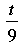SEARCH HOMEMath Central Quandaries & QueriesQuestion from David, a parent: Hello My daughter has two questions that we could use some help with. First ? Four less than three times a number is 14 Second? The quotient of -15 and w Thank youDavid,

How would you calculate four less than three times eight? I would find three times eight, 3 × 8 = 24, and then subtract four. So four less than three times eight is

3 × 8 - 4 = 28 - 4 = 24.

In your daughter's problem she doesn't know the number where I used eight, she just knows that whatever the number is, if you multiply it by 3 and then subtract 4 you get 14. To write a mathematical expression for this, rather than use words we use a letter to stand for the unknown number. So if I use the letter x to stand for the unknown number then the statement in the first problem is

four less than three times x is 14

which written as an equation is

3 × x - 4 = 14.

In the second problem the word quotient signifies division. I am not sure how your daughter's teacher writes division, for example

the quotient of t and 9

could be written

t/9

or

t ÷ 9

or.

PennyMath Central is supported by the University of Regina and The Pacific Institute for the Mathematical Sciences.